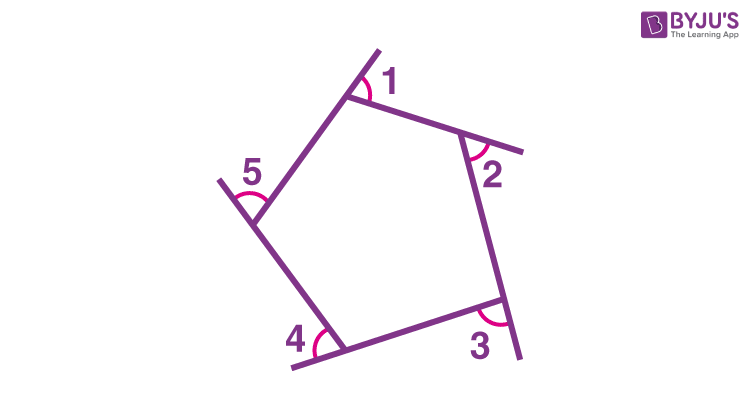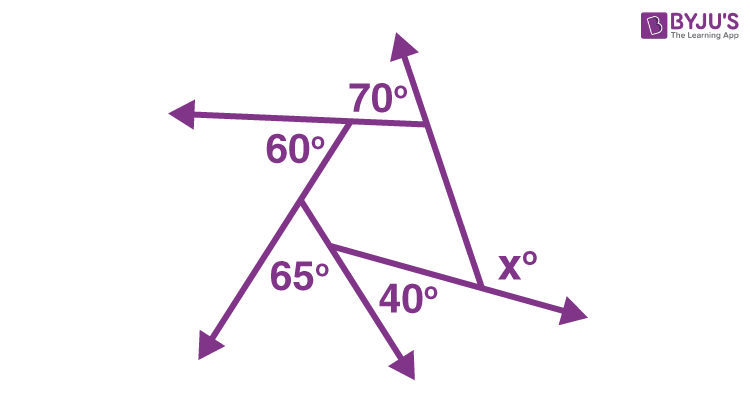# Exterior Angles Of A Polygon

Exterior angles of a polygon are formed when by one of its side and extending the other side. The sum of all the exterior angles in a polygon is equal to 360 degrees. You are already aware of the term polygon. A polygon is a flat figure that is made up of three or more line segments and is enclosed. The line segments are called the sides and the point where two sides meet is called the vertex of the polygon. The pair of sides that meet at the same vertex are called adjacent sides. An angle at one of the vertices is called the interior angle. The internal and exterior angles at each vertex varies for all types of polygons.  Now, let us learn in detail the concept of its exterior angles.

## What are Exterior Angles?

An exterior angle is an angle which is formed by one of the sides of any closed shape structure such as polygon and the extension of its adjacent side. See the figure below, where a five-sided polygon or pentagon is having 5 vertexes. The exterior angles of this pentagon are formed by extending its adjacent sides.• They are formed on the outside or exterior of the polygon.
• The sum of an interior angle and its corresponding exterior angle is always 180 degrees since they lie on the same straight line.
• In the figure, angles 1, 2, 3, 4 and 5 are the exterior angles of the polygon.

Note: Exterior angles of a regular polygon are equal in measure.

### Sum of the Exterior Angles of a Polygon

Let us say you start travelling from the vertex at angle 1. You go in a clockwise direction, make turns through angles 2, 3, 4 and 5 and come back to the same vertex. You covered the entire perimeter of the polygon and in fact, made one complete turn in the process. One complete turn is equal to 360 degrees. Thus, it can be said that ∠1, ∠2, ∠3, ∠4 and ∠5 sum up to 360 degrees.

Hence, the sum of the measures of the exterior angles of a polygon is equal to 360 degrees, irrespective of the number of sides in the polygons.

### Polygon Exterior Angle Sum Theorem

If a polygon is a convex polygon, then the sum of its exterior angles (one at each vertex) is equal to 360 degrees. Let us prove this theorem:

Proof: Consider a polygon with n number of sides or an n-gon. The sum of its exterior angles is N.

For any closed structure, formed by sides and vertex, the sum of the exterior angles is always equal to the sum of linear pairs and sum of interior angles. Therefore,

N = 180n – 180(n-2)

N = 180n – 180n + 360

N = 360

Hence, we got the sum of exterior angles of n vertex equal to 360 degrees.

## Video Lesson on Angle sum and exterior angle property### Exterior Angles Examples

Example 1: In the given figure, find the value of x.Solution: We know that the sum of exterior angles of a polygon is 360 degrees.

Thus, 70° + 60° + 65° + 40° + x = 360°

235° + x = 360°

X = 360° – 235° = 125°

Example 2: Identify the type of regular polygon whose exterior angle measures 120 degrees.

Solution: Since the polygon is regular, the measure of all the interior angles is the same. Therefore, all its exterior angles measure the same as well, that is, 120 degrees.

Since the sum of exterior angles is 360 degrees and each one measures 120 degrees, we have,

Number of angles = 360/120 = 3

Since the polygon has 3 exterior angles, it has 3 sides. Hence it is an equilateral triangle.

1. The polygon in which the sum of all the exterior angles is equal to the sum of all the interior angles is called ______________.

• The polygon in which the sum of all the exterior angles is equal to the sum of all the interior angles is called Quadrilateral.

• Is rhombus and parallelogram is also quadrilateral

• Both rhombus and parallelogram are closed shape that has four sides. Thus, both are quadrilaterals.